1.6 Absolute value functions  (Page 5/7)

 Page 5 / 7

Solve $\text{\hspace{0.17em}}-2|k-4|\le -6.$

$k\le 1\text{\hspace{0.17em}}$ or $\text{\hspace{0.17em}}k\ge 7;\text{\hspace{0.17em}}$ in interval notation, this would be $\text{\hspace{0.17em}}\left(-\infty ,1\right]\cup \left[7,\infty \right)$

Key concepts

• The absolute value function is commonly used to measure distances between points. See [link] .
• Applied problems, such as ranges of possible values, can also be solved using the absolute value function. See [link] .
• The graph of the absolute value function resembles a letter V. It has a corner point at which the graph changes direction. See [link] .
• In an absolute value equation, an unknown variable is the input of an absolute value function.
• If the absolute value of an expression is set equal to a positive number, expect two solutions for the unknown variable. See [link] .
• An absolute value equation may have one solution, two solutions, or no solutions. See [link] .
• An absolute value inequality is similar to an absolute value equation but takes the form It can be solved by determining the boundaries of the solution set and then testing which segments are in the set. See [link] .
• Absolute value inequalities can also be solved graphically. See [link] .

Verbal

How do you solve an absolute value equation?

Isolate the absolute value term so that the equation is of the form $\text{\hspace{0.17em}}|A|=B.\text{\hspace{0.17em}}$ Form one equation by setting the expression inside the absolute value symbol, $\text{\hspace{0.17em}}A,\text{\hspace{0.17em}}$ equal to the expression on the other side of the equation, $\text{\hspace{0.17em}}B.\text{\hspace{0.17em}}$ Form a second equation by setting $\text{\hspace{0.17em}}A\text{\hspace{0.17em}}$ equal to the opposite of the expression on the other side of the equation, $\text{\hspace{0.17em}}-B.\text{\hspace{0.17em}}$ Solve each equation for the variable.

How can you tell whether an absolute value function has two x -intercepts without graphing the function?

When solving an absolute value function, the isolated absolute value term is equal to a negative number. What does that tell you about the graph of the absolute value function?

The graph of the absolute value function does not cross the $\text{\hspace{0.17em}}x$ -axis, so the graph is either completely above or completely below the $\text{\hspace{0.17em}}x$ -axis.

How can you use the graph of an absolute value function to determine the x -values for which the function values are negative?

How do you solve an absolute value inequality algebraically?

First determine the boundary points by finding the solution(s) of the equation. Use the boundary points to form possible solution intervals. Choose a test value in each interval to determine which values satisfy the inequality.

Algebraic

Describe all numbers $\text{\hspace{0.17em}}x\text{\hspace{0.17em}}$ that are at a distance of 4 from the number 8. Express this using absolute value notation.

Describe all numbers $\text{\hspace{0.17em}}x\text{\hspace{0.17em}}$ that are at a distance of $\text{\hspace{0.17em}}\frac{1}{2}\text{\hspace{0.17em}}$ from the number −4. Express this using absolute value notation.

$\text{\hspace{0.17em}}|x+4|=\frac{1}{2}\text{\hspace{0.17em}}$

Describe the situation in which the distance that point $\text{\hspace{0.17em}}x\text{\hspace{0.17em}}$ is from 10 is at least 15 units. Express this using absolute value notation.

Find all function values $\text{\hspace{0.17em}}f\left(x\right)\text{\hspace{0.17em}}$ such that the distance from $\text{\hspace{0.17em}}f\left(x\right)\text{\hspace{0.17em}}$ to the value 8 is less than 0.03 units. Express this using absolute value notation.

$|f\left(x\right)-8|<0.03$

For the following exercises, solve the equations below and express the answer using set notation.

$|x+3|=9$

$|6-x|=5$

$\left\{1,11\right\}$

$|5x-2|=11$

$|4x-2|=11$

$\left\{\frac{9}{4},\text{\hspace{0.17em}}\text{\hspace{0.17em}}\text{\hspace{0.17em}}\text{\hspace{0.17em}}\text{\hspace{0.17em}}\frac{13}{4}\right\}$

$2|4-x|=7$

$3|5-x|=5$

$\left\{\frac{10}{3},\text{\hspace{0.17em}}\text{\hspace{0.17em}}\text{\hspace{0.17em}}\text{\hspace{0.17em}}\text{\hspace{0.17em}}\frac{20}{3}\right\}$

$3|x+1|-4=5$

$5|x-4|-7=2$

$\left\{\frac{11}{5},\text{\hspace{0.17em}}\text{\hspace{0.17em}}\text{\hspace{0.17em}}\text{\hspace{0.17em}}\text{\hspace{0.17em}}\frac{29}{5}\right\}$

$0=-|x-3|+2$

$2|x-3|+1=2$

$\left\{\frac{5}{2},\frac{7}{2}\right\}$

$|3x-2|=7$

$|3x-2|=-7$

No solution

$|\frac{1}{2}x-5|=11$

$|\frac{1}{3}x+5|=14$

$\left\{-57,27\right\}$

$-|\frac{1}{3}x+5|+14=0$

For the following exercises, find the x - and y -intercepts of the graphs of each function.

$f\left(x\right)=2|x+1|-10$

$\left(0,-8\right);\text{\hspace{0.17em}}\left(-6,0\right),\text{\hspace{0.17em}}\left(4,0\right)$

$f\left(x\right)=4|x-3|+4$

$f\left(x\right)=-3|x-2|-1$

$\left(0,-7\right);\text{\hspace{0.17em}}$ no $\text{\hspace{0.17em}}x$ -intercepts

$f\left(x\right)=-2|x+1|+6$

For the following exercises, solve each inequality and write the solution in interval notation.

$|x-2|>10$

$\left(-\infty ,-8\right)\cup \left(12,\infty \right)$

$2|v-7|-4\ge 42$

$|3x-4|\le 8$

$\frac{-4}{3}\le x\le 4$

$|x-4|\ge 8$

$|3x-5|\ge 13$

$\left(-\infty ,-\frac{8}{3}\right]\cup \left[6,\infty \right)$

$|3x-5|\ge -13$

$|\frac{3}{4}x-5|\ge 7$

$\left(-\infty ,-\frac{8}{3}\right]\cup \left[16,\infty \right)$

$|\frac{3}{4}x-5|+1\le 16$

Graphical

For the following exercises, graph the absolute value function. Plot at least five points by hand for each graph.

$y=|x-1|$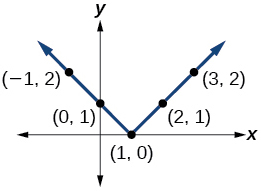$y=|x+1|$

$y=|x|+1$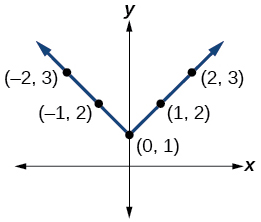For the following exercises, graph the given functions by hand.

$y=|x|-2$

$y=-|x|$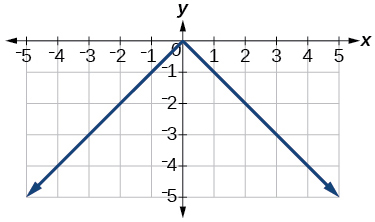$y=-|x|-2$

$y=-|x-3|-2$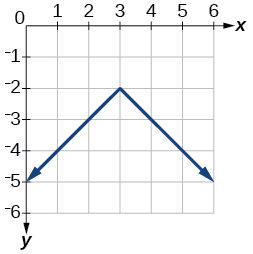$f\left(x\right)=-|x-1|-2$

$f\left(x\right)=-|x+3|+4$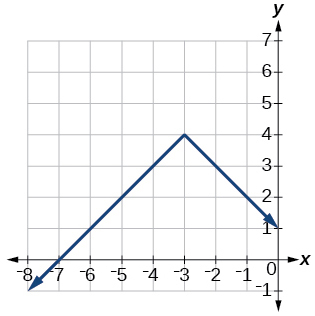$f\left(x\right)=2|x+3|+1$

$f\left(x\right)=3|x-2|+3$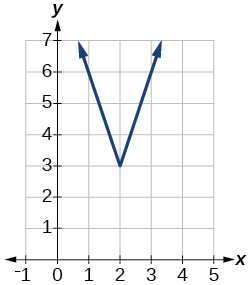$f\left(x\right)=|2x-4|-3$

$f\left(x\right)=|3x+9|+2$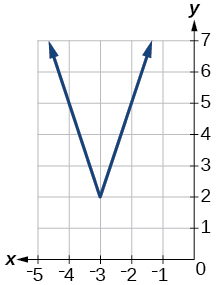$f\left(x\right)=-|x-1|-3$

$f\left(x\right)=-|x+4|-3$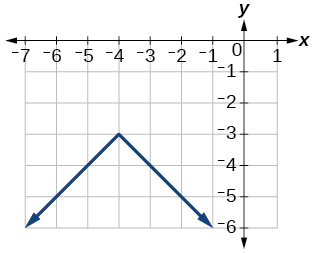$f\left(x\right)=\frac{1}{2}|x+4|-3$

Technology

Use a graphing utility to graph $f\left(x\right)=10|x-2|$ on the viewing window $\left[0,4\right].$ Identify the corresponding range. Show the graph.

range: $\text{\hspace{0.17em}}\left[0,20\right]$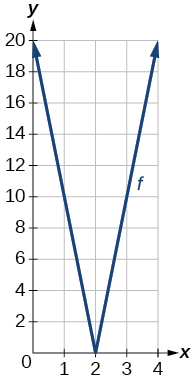Use a graphing utility to graph $\text{\hspace{0.17em}}f\left(x\right)=-100|x|+100\text{\hspace{0.17em}}$ on the viewing window $\text{\hspace{0.17em}}\left[-5,5\right].\text{\hspace{0.17em}}$ Identify the corresponding range. Show the graph.

For the following exercises, graph each function using a graphing utility. Specify the viewing window.

$f\left(x\right)=-0.1|0.1\left(0.2-x\right)|+0.3$

$x\text{-}$ intercepts: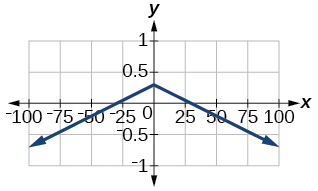$f\left(x\right)=4×{10}^{9}|x-\left(5×{10}^{9}\right)|+2×{10}^{9}$

Extensions

For the following exercises, solve the inequality.

$|-2x-\frac{2}{3}\left(x+1\right)|+3>-1$

$\left(-\infty ,\infty \right)$

If possible, find all values of $a$ such that there are no $x\text{-}$ intercepts for $f\left(x\right)=2|x+1|+a.$

If possible, find all values of $\text{\hspace{0.17em}}a\text{\hspace{0.17em}}$ such that there are no $\text{\hspace{0.17em}}y$ -intercepts for $\text{\hspace{0.17em}}f\left(x\right)=2|x+1|+a.$

There is no solution for $\text{\hspace{0.17em}}a\text{\hspace{0.17em}}$ that will keep the function from having a $\text{\hspace{0.17em}}y$ -intercept. The absolute value function always crosses the $\text{\hspace{0.17em}}y$ -intercept when $\text{\hspace{0.17em}}x=0.$

Real-world applications

Cities A and B are on the same east-west line. Assume that city A is located at the origin. If the distance from city A to city B is at least 100 miles and $\text{\hspace{0.17em}}x\text{\hspace{0.17em}}$ represents the distance from city B to city A, express this using absolute value notation.

The true proportion $\text{\hspace{0.17em}}p\text{\hspace{0.17em}}$ of people who give a favorable rating to Congress is 8% with a margin of error of 1.5%. Describe this statement using an absolute value equation.

$|p-0.08|\le 0.015$

Students who score within 18 points of the number 82 will pass a particular test. Write this statement using absolute value notation and use the variable $\text{\hspace{0.17em}}x\text{\hspace{0.17em}}$ for the score.

A machinist must produce a bearing that is within 0.01 inches of the correct diameter of 5.0 inches. Using $\text{\hspace{0.17em}}x\text{\hspace{0.17em}}$ as the diameter of the bearing, write this statement using absolute value notation.

$|x-5.0|\le 0.01$

The tolerance for a ball bearing is 0.01. If the true diameter of the bearing is to be 2.0 inches and the measured value of the diameter is $\text{\hspace{0.17em}}x\text{\hspace{0.17em}}$ inches, express the tolerance using absolute value notation.

I've run into this: x = r*cos(angle1 + angle2) Which expands to: x = r(cos(angle1)*cos(angle2) - sin(angle1)*sin(angle2)) The r value confuses me here, because distributing it makes: (r*cos(angle2))(cos(angle1) - (r*sin(angle2))(sin(angle1)) How does this make sense? Why does the r distribute once
How can you tell what type of parent function a graph is ?
generally by how the graph looks and understanding what the base parent functions look like and perform on a graph
William
if you have a graphed line, you can have an idea by how the directions of the line turns, i.e. negative, positive, zero
William
y=x will obviously be a straight line with a zero slope
William
y=x^2 will have a parabolic line opening to positive infinity on both sides of the y axis vice versa with y=-x^2 you'll have both ends of the parabolic line pointing downward heading to negative infinity on both sides of the y axis
William
y=x will be a straight line, but it will have a slope of one. Remember, if y=1 then x=1, so for every unit you rise you move over positively one unit. To get a straight line with a slope of 0, set y=1 or any integer.
Aaron
yes, correction on my end, I meant slope of 1 instead of slope of 0
William
what is f(x)=
I don't understand
Joe
Typically a function 'f' will take 'x' as input, and produce 'y' as output. As 'f(x)=y'. According to Google, "The range of a function is the complete set of all possible resulting values of the dependent variable (y, usually), after we have substituted the domain."
Thomas
Sorry, I don't know where the "Â"s came from. They shouldn't be there. Just ignore them. :-)
Thomas
Darius
Thanks.
Thomas
Â
Thomas
It is the Â that should not be there. It doesn't seem to show if encloses in quotation marks. "Â" or 'Â' ... Â
Thomas
Now it shows, go figure?
Thomas
what is this?
i do not understand anything
unknown
lol...it gets better
Darius
I've been struggling so much through all of this. my final is in four weeks 😭
Tiffany
this book is an excellent resource! have you guys ever looked at the online tutoring? there's one that is called "That Tutor Guy" and he goes over a lot of the concepts
Darius
thank you I have heard of him. I should check him out.
Tiffany
is there any question in particular?
Joe
I have always struggled with math. I get lost really easy, if you have any advice for that, it would help tremendously.
Tiffany
Sure, are you in high school or college?
Darius
Hi, apologies for the delayed response. I'm in college.
Tiffany
how to solve polynomial using a calculator
So a horizontal compression by factor of 1/2 is the same as a horizontal stretch by a factor of 2, right?
The center is at (3,4) a focus is at (3,-1), and the lenght of the major axis is 26
The center is at (3,4) a focus is at (3,-1) and the lenght of the major axis is 26 what will be the answer?
Rima
I done know
Joe
What kind of answer is that😑?
Rima
I had just woken up when i got this message
Joe
Rima
i have a question.
Abdul
how do you find the real and complex roots of a polynomial?
Abdul
@abdul with delta maybe which is b(square)-4ac=result then the 1st root -b-radical delta over 2a and the 2nd root -b+radical delta over 2a. I am not sure if this was your question but check it up
Nare
This is the actual question: Find all roots(real and complex) of the polynomial f(x)=6x^3 + x^2 - 4x + 1
Abdul
@Nare please let me know if you can solve it.
Abdul
I have a question
juweeriya
hello guys I'm new here? will you happy with me
mustapha
The average annual population increase of a pack of wolves is 25.
how do you find the period of a sine graph
Period =2π if there is a coefficient (b), just divide the coefficient by 2π to get the new period
Am
if not then how would I find it from a graph
Imani
by looking at the graph, find the distance between two consecutive maximum points (the highest points of the wave). so if the top of one wave is at point A (1,2) and the next top of the wave is at point B (6,2), then the period is 5, the difference of the x-coordinates.
Am
you could also do it with two consecutive minimum points or x-intercepts
Am
I will try that thank u
Imani
Case of Equilateral Hyperbola
ok
Zander
ok
Shella
f(x)=4x+2, find f(3)
Benetta
f(3)=4(3)+2 f(3)=14
lamoussa
14
Vedant
pre calc teacher: "Plug in Plug in...smell's good" f(x)=14
Devante
8x=40
Chris
Explain why log a x is not defined for a < 0
the sum of any two linear polynomial is what
Momo
how can are find the domain and range of a relations
the range is twice of the natural number which is the domain
Morolake
A cell phone company offers two plans for minutes. Plan A: $15 per month and$2 for every 300 texts. Plan B: $25 per month and$0.50 for every 100 texts. How many texts would you need to send per month for plan B to save you money?
6000
Robert
more than 6000
Robert
For Plan A to reach $27/month to surpass Plan B's$26.50 monthly payment, you'll need 3,000 texts which will cost an additional \$10.00. So, for the amount of texts you need to send would need to range between 1-100 texts for the 100th increment, times that by 3 for the additional amount of texts...
Gilbert
...for one text payment for 300 for Plan A. So, that means Plan A; in my opinion is for people with text messaging abilities that their fingers burn the monitor for the cell phone. While Plan B would be for loners that doesn't need their fingers to due the talking; but those texts mean more then...
GilbertByByBy Anonymous UserBy Abishek DevarajBy Sebastian Sieczko...ByBy